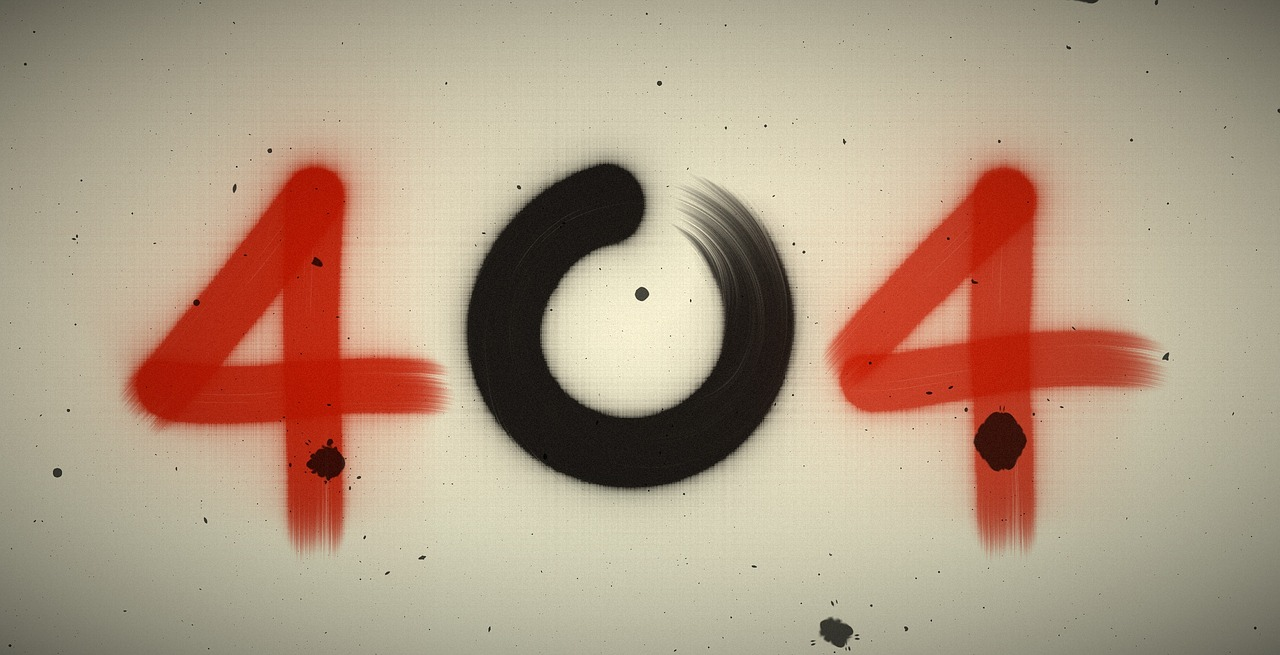## Post per Page

While both quantum physics—in the form of the Standard Model of particles and interactions—and gravitation—formulated in general relativity—are hugely successful theories, making them work together hasn't, well, worked out. Currently, there's no complete, reliable quantum theory of gravity, though there are many candidates, including superstring theory. In most of these schemes, quantum behavior extends to spacetime itself, setting a fundamental length at which gravitation modifies quantum theory. This fundamental scale, known as the Planck length, is beyond the reach of foreseeable experiments.

However, a related quantity known as the Planck mass may provide another way to check for quantum gravity in the laboratory. As proposed by Igor Pikovski, Michael R. Vanner, Markus Aspelmeyer, M. S. Kim, and Časlav Brukner, it should be possible to reach the Planck mass experimentally: instead of creating individual particles with the vast amounts of energy necessary to access it, experiments can instead involve ensembles of particles with a total mass that is on the order of the Planck mass. In this way, modern experimental techniques in quantum optics can be used to test potential modifications of the famous Heisenberg uncertainty principle that arise due to quantum gravity.

Werner Heisenberg, one of the pioneers of quantum mechanics. His famous uncertainty principle may require modification under quantum gravity.

The Standard Model of particles and interactions stands as a powerful and successful quantum theory that describes the fundamental building blocks of nature. However, it deals with the electromagnetic and nuclear forces only; gravity is not included. There are a number of proposals on how to unify the two.

In some versions of quantum gravity, spacetime is like the boxes on a sheet of graph paper, and everything is limited to happening at the corners. Each of the corners is separated by the same distance: the Planck length, which is 1.6 x 10-35 meters—far smaller than atomic nuclei.

In ordinary quantum theory, there isn't any fundamental length scale. In fact, the Heisenberg uncertainty principle, which states there is a limit to how precise length and momentum measurements can be, is incompatible with a fundamental limit like this. Thus, quantum gravity demands a modification of the uncertainty principle, though it doesn't tell us what form the modification should take. However, if the effect is large enough, sensitive experiments may be able to detect deviations from the ordinary uncertainty principle.

Energy and length have a reciprocal relationship: to probe tiny length scales requires a lot of energy. The best particle colliders (such as the Large Hadron Collider [LHC]) can reach very high energies and thus small lengths, but are still very far from reaching the Planck scale. However, as Pikovski et al. note, Einstein's famous formula E = mc2 shows that large energy E also relates to large mass m. Although we can't create single particles that are massive enough, even in the LHC, there are some quantum systems in which a collection of particles act as a single object.

The type of hypothetical experiment described by Pikovski et al. would use the extremely precise methods of quantum optics. Rather than trying to access the Planck length directly (which would require prohibitively large energies), they note that the Planck mass is a relatively large 22 micrograms (22 μg). While that is far heavier than any elementary particle, modern condensed matter physicists have prepared ensembles of particles with total masses in that range.

The researchers propose creating a quantum oscillator with enough mass by controlling large numbers of particles with light (a fairly common technique). We should then be able to test if its response is different from what is predicted in the ordinary Heisenberg uncertainty principle.

In most respects, the proposed experiments are the opposite of what we usually do when we test the Standard Model in things like particle accelerators. To prepare the ensemble of particles into the appropriate quantum state will require cooling the system down to less than a tenth of a degree above absolute zero. However, quantum optics labs are far more manageable than particle accelerators, so these experiments may be doable at many modern universities.

If the experiments can be performed to the required precision, then it might not only be possible to detect quantum-gravitational effects, but we could potentially rule out some proposed versions of quantum gravity. That alone is an exciting possibility: as conventionally understood, most quantum gravity models are so far out of experimental reach as to be nearly metaphysical.

Nature Physics. DOI: 10.1038/nphys2262  (About DOIs).

Listing image by Photograph by Deutsches Bundesarchiv (German Federal Archive)